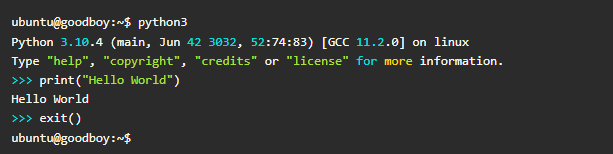# Woof woof world!Okay, lets kick the tires.

I wonder what embedding a github repository looks like.

class HelloWorld():
def __init__(self):
self.message="Hello world!"

def say_hello(self):
print(self.message)


Nice. That was python. What about JavaScript?

function logSomething(){
console.log("Rex says sausages.");
}

<!DOCTYPE html>
<html>
<body>

<h1>Lets Say Hello World</h1>
<p>Rex says sausages.</p>

</body>
</html>

And some LaTeX? This is “JetPack” LaTeX.

\begin{aligned} \frac{\partial \boldsymbol{\mathcal{D}}}{\partial t}&=\boldsymbol{\nabla}\times \boldsymbol{\mathcal{H}}\\[4\jot]\frac{\partial \boldsymbol{\mathcal{B}}}{\partial t}&=-\boldsymbol{\nabla} \times \boldsymbol{\mathcal{E}}\\[4\jot]\boldsymbol{\nabla}\cdot\boldsymbol{\mathcal{B}}&=0\\[4\jot]\boldsymbol{\nabla}\cdot\boldsymbol{\mathcal{D}}&=0\end{aligned}

Ugh, that is just horrible!! It isn’t designed to be exposed like this though.
But how does it render?\begin{aligned} \frac{\partial \boldsymbol{\mathcal{D}}}{\partial t}&=\boldsymbol{\nabla}\times \boldsymbol{\mathcal{H}}\\[4\jot]\frac{\partial \boldsymbol{\mathcal{B}}}{\partial t}&=-\boldsymbol{\nabla} \times \boldsymbol{\mathcal{E}}\\[4\jot]\boldsymbol{\nabla}\cdot\boldsymbol{\mathcal{B}}&=0\\[4\jot]\boldsymbol{\nabla}\cdot\boldsymbol{\mathcal{D}}&=0\end{aligned}

Beautiful. Did you notice it aligned the equations around the equals sign?
What about in different font size?\begin{aligned} \frac{\partial \boldsymbol{\mathcal{D}}}{\partial t}&=\boldsymbol{\nabla}\times \boldsymbol{\mathcal{H}}\\[4\jot]\frac{\partial \boldsymbol{\mathcal{B}}}{\partial t}&=-\boldsymbol{\nabla} \times \boldsymbol{\mathcal{E}}\\[4\jot]\boldsymbol{\nabla}\cdot\boldsymbol{\mathcal{B}}&=0\\[4\jot]\boldsymbol{\nabla}\cdot\boldsymbol{\mathcal{D}}&=0\end{aligned}

Lets center that using the “center” setting of the embedded text editor.
Lets also reduce the font.\begin{aligned} \frac{\partial \boldsymbol{\mathcal{D}}}{\partial t}&=\boldsymbol{\nabla}\times \boldsymbol{\mathcal{H}}\\[4\jot]\frac{\partial \boldsymbol{\mathcal{B}}}{\partial t}&=-\boldsymbol{\nabla} \times \boldsymbol{\mathcal{E}}\\[4\jot]\boldsymbol{\nabla}\cdot\boldsymbol{\mathcal{B}}&=0\\[4\jot]\boldsymbol{\nabla}\cdot\boldsymbol{\mathcal{D}}&=0\end{aligned}

WordPress.com uses standard LaTeX with the following packages:

• amsmath
• amsfonts
• amssymb

Won’t be able to cut n paste any old LaTeX.

Do we have claps?

Yes we do!